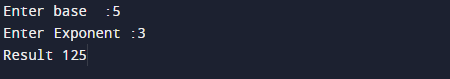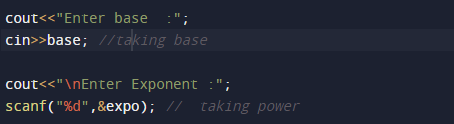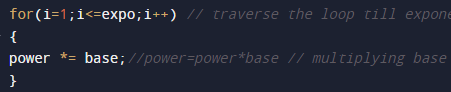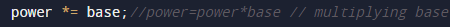# C++ Program to Calculate Power of a Number

In this tutorial you will learn about the C++ Program to Calculate Power of a Number and its application with practical example.

In this tutorial, we will learn to create a C++ program that will find the Power of a given number  using C++ programming.

## Prerequisites

Before starting with this tutorial we assume that you are best aware of the following C programming topics:

• Operators.
• Precedence.
• Looping statements.
• Basic input/output functions.
• Basic c++ programming.

## Calculating Power of a Number.

let’s have a look Logic behind to finding the power of any number  using  C++ programming.

## Example

Input:
take  value at  base : 3
take  value at exponent : 3
So power of any number basen given as base*base*…..*base (n-times). Here base is called base and n is called the exponent.Here we will write a C++ program to find the power of a given number.

## Program to find power of any number.

In this program we will find power of any given number using for loop. We would first declared and initialized the required variables. Next, we would prompt user to input the number and power of number .Then we will find the power of given number.

## OutputPoint to remember : The above program will works only if the Power is a positive integer (number).

In the above program, we have first declared and initialized a set variables required in the program.

• base = it hold the value of base
• expo = it will hold the power
• power= it will hold the result.

Let see the logic of program step by step.

1. Input base and exponents from user, and put  it in two variables  base and expo.2. Initialize variable that will store power to one (power=1).
3.within the loop value of i start from 1 to expo, increment loop  by 1 in each iteration. The structure  look like.4.For every iteration the value of  power  multiply with base.5.Finally after iteration we get the answer in variable Power.

In this tutorial we understand how to write a program without using Power Function In C++ to calculate power of a  given number.In this tutorial we have learn about the C++ Program to Calculate Power of a Number and its application with practical example. I hope you will like this tutorial.